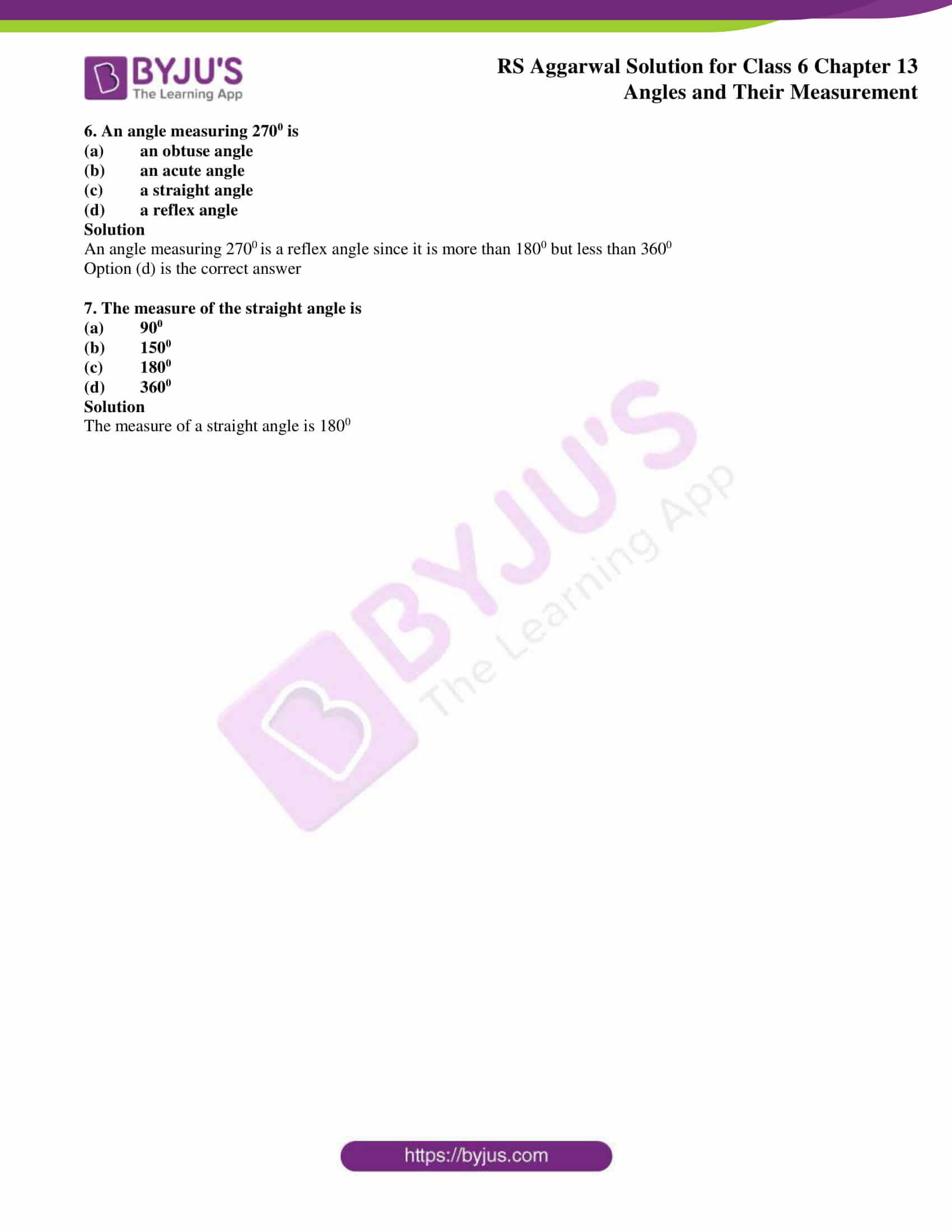# RS Aggarwal Solutions for Class 6 Chapter 13 Angles and their Measurement Exercise 13D

RS Aggarwal Solutions are prepared with the primary aim of helping students with their exam preparation. The fourth exercise of chapter 13 has objective type problems which are important from exam point of view. The exercise 13D covers the entire syllabus and has important concepts and formulas highlighted to make them easy for the students. Brief knowledge about the topics covered in RS Aggarwal Solutions for Class 6 Chapter 13 Angles and their Measurement Exercise 13D can be downloaded here in PDF format.

## Download PDF of RS Aggarwal Solutions for Class 6 Chapter 13 Angles and their Measurement Exercise 13D### Access answers to Maths RS Aggarwal Solutions for Class 6 Chapter 13 Angles and their Measurement Exercise 13D

Objective Questions

Mark () against the correct answer in each of the following:

1. Where does the vertex of an angle lie?

(a) In its interior

(b) In its exterior

(c) On the angle

(d) None of these

Solution

The common initial point is known as vertex of the angle. Hence, it lies on the angle

Option (c) is the correct answer

2. The figure formed by two rays with the same initial point is called

(a) a ray

(b) a line

(c) an angle

(d) none of these

Solution

The figure formed by the angle with the same initial point is called an angle

Option (c) is the correct answer

3. An angle measuring 1800 is called

(a) a complete angle

(b) a reflex angle

(c) a straight angle

(d) none of this

Solution

An angle measuring 1800 is a straight line

Option (c) is the correct answer

4. An angle measuring 900 is called

(a) a straight angle

(b) a right angle

(c) a complete angle

(d) a reflex angle

Solution

An angle measuring 900 is called a right angle

Option (b) is the correct answer

5. An angle measuring 910 is

(a) an acute angle

(b) an obtuse angle

(c) a reflex angle

(d) none of this

Solution

An angle measuring 910 is called an obtuse angle which is more than 900 but less than 1800

Option (b) is the correct answer

6. An angle measuring 2700 is

(a) an obtuse angle

(b) an acute angle

(c) a straight angle

(d) a reflex angle

Solution

An angle measuring 2700 is a reflex angle since it is more than 1800 but less than 3600

Option (d) is the correct answer

7. The measure of the straight angle is

(a) 900

(b) 1500

(c) 1800

(d) 3600

Solution

The measure of a straight angle is 1800

### Access other exercises of RS Aggarwal Solutions for Class 6 Chapter 13 Angles and their Measurement

Exercise 13C Solutions